# Chemistry

Some of the content of this guide was modeled after a guide originally created by Openstax and has been adapted for the GPRC Learning Commons in April 2021.Percent Composition

The percent composition of an element in a compound is calculated using the formula.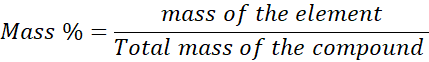Example 1

What is the percent composition of FeSO4?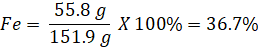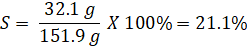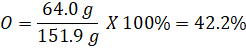The total percent composition adds up to 100% (36.7% + 21.1 % + 42.2% = 100%)

Determination of Empirical Formulas

The empirical formula is used to show the relative ratios of various atoms of a compound.

The masses of each element in a compound can be measured using experiments with that the empirical formula is derived as shown in the example below.

Find the empirical formula of a compound that contains 38.7% C, 16.2% H, and 45.1% N.

Step 1:

Assume 100 g of the compound then the number of moles of each element can be calculated as follows.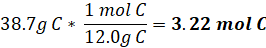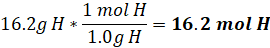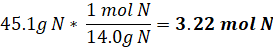Step 2: Determining mole ratio

C3.22H16.2N3.22

Step 3 : Divide all the moles by the smallest one found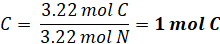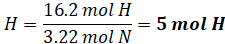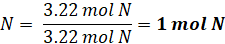Step 4: The empirical formula now can be written as follows

C1H5N1 = CH5N

Derivation of Molecular Formulas

Ethylene glycol has 38.7% C, 9.75% H, and Oxygen. Its molecular weight is 62.07g. Find the molecular formula of ethylene glycol.

Step 1

The amount of oxygen in the compound can be found using the following method since the total percent composition is equal to 100%

O = 100% - (38.7% + 9.75%) = 51.55%

Step 2

Assume 100 g of the compound then the number of moles of each element can be calculated as follows.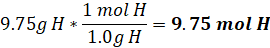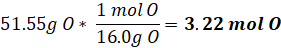Step 3

Divide all the moles by the smallest one found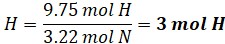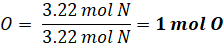Then the empirical formula becomes CH3O

Step 4

Calculating the ratio of molecular weight to empirical forumal weight

Molecular weight = 62.07g

Empirical formula weight = (1*12.0g)+(3*1.0g)+(1*16.0g) = 31.0g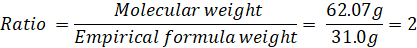Step 5

Multiply the mols of empirical formula by the ratio calculated in step 4

Then the molecular formula becomes C2H6O2